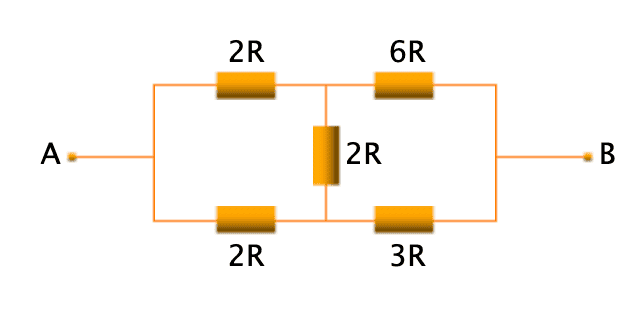# Find the equivalent resistance between two points in terms of R

• valhakla

#### valhakla

Homework Statement
Find thr equivalent resistance through two point A and B
Relevant Equations
R series = R1 + R2
R parallel = R1*R2/R1+R2#### Attachments

According to our rules, to receive help, you need to show some credible effort towards answering the question(s). How about telling us what you do know and how you would approach this problem?

I attempted to solve the problem using the formulas I have provided above, but my answers are not matching, also I tried to search for similar problems in the internet but found nothing

OK, but "I tried and didn't get anywhere" is not sufficient attempt at a solution. Please post what you tried and what you got. We would prefer that you use LaTeX which is to learn. Click the link "LaTeX guide", lower left, to see how to do it.

New user is reminded that they always need to show their best efforts on schoolwork problems before we can offer tutorial help.
OK, but "I tried and didn't get anywhere" is not sufficient attempt at a solution. Please post what you tried and what you got. We would prefer that you use LaTeX which is to learn. Click the link "LaTeX guide", lower left, to see how to do it.
Thank you, I will learn how to do it. I am just learning and new here, so would appreciate solution for my problem, the problem seems very unique for me as it's really challenging for me to determine whether the resistors are in parallel or in series, otherwise I wouldn't post it here.

Thank you, I will learn how to do it. I am just learning and new here, so would appreciate solution for my problem, the problem seems very unique for me as it's really challenging for me to determine whether the resistors are in parallel or in series, otherwise I wouldn't post it here.
We don't supply solutions, only hints and you must make and show an effort to use them. So here are some.

What are the criteria for resistors being in series?
What are the criteria for resistors being in parallel?
Do you see any resistor combination in this circuit that satisfies these criteria? If so identify them.